# Combining Like Terms

in hive-122481 •  17 days ago

Hi there. This math post is on the algebra topic of combining like terms. In the province of Ontario in Canada, this particular topic is typically taught in grade nine (first year high school). This could also be taught in grade eight.

Math text and symbols from using QuickLaTeX.com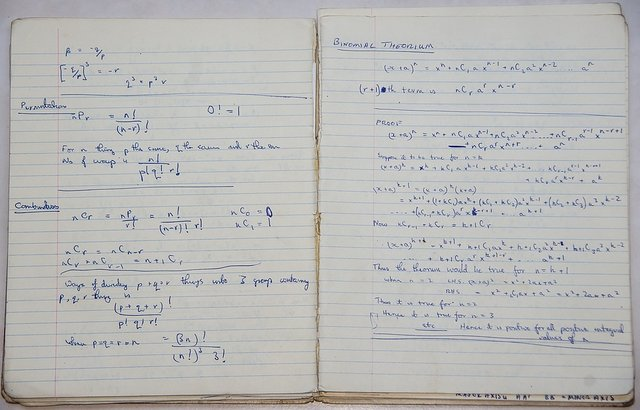Pixabay Image Source

## Topics

• Variable Terms Vs Constants Vs Coefficients
• Examples Of Combining Like Terms
• Examples Of Solving Equations With Like Terms
• Practice Problems
• Solutions To Practice Problems

### Variable Terms Vs Constants Vs Coefficients

Terminology lessons are boring sometimes but they are needed.

A constant refers to a number on its own. There are no variables like x, y, z associated with this number. Examples include 2, 10 8.5, pi, 8/5, etc.

A variable represents an unknown quantity. Variable terms includes numbers and variables such as 2x, 3y, 10xy and so on. In a variable term, the number in front of the variable part is called the coefficient.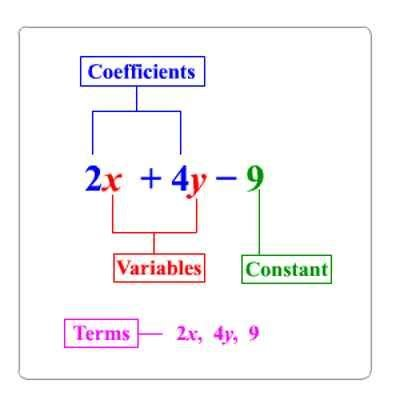Duckduckgo Search Image

An algebraic expression consists of various numbers and unknown quantities. These expressions do not contain an equal sign so they don't have another "side". Expressions also contain terms which refer to an unknown quantity. Terms are separated with plus and minus signs.

Examples of expressions include:

• x + y
• 2x + 1
• 100 - x - 3y
• 2xyz - q + 7r

The expression examples above were all different in the sense that the terms for each of a different type. For expression 100 -x -3y could not be simplified further as there is no other constant number, there is not other term with an x and there is no other term with a variable y.

If there was an expression such as `2x + 7 - x`, this can be reduced to `7 + x`. The simplifying step involves leaving the +7 alone as it has no variable x and doing 2x minus x (or 2x plus negative x).

If the previous example is confusing, consider this example. Suppose there are 4 apples from Monday, 3 bananas from Tuesday, 8 apples from Wednesday and 1 banana eaten on Thursday. What does the fruit basket look like on Friday? Adding up all the apples gives 4 + 8 = 12 and the bananas count would be 3 - 1 = 2 bananas. Friday's fruit basket inventory would be 12 apples and 2 bananas. The key thing is here is to add/subtract apples with apples and add/subtract bananas with bananas.Pixabay Image Source

### Examples Of Combining Like Terms

Here are some worked out examples of combining like terms.

Example One

The expression -2x + 7x -1 simplifies to 5x - 1. This is from -2x + 7x = 5x. The negative 1 is left alone.

Example Two

What if we want to simplify expressions that contain higher exponents? Let's take a look.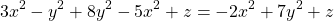Example Three

This next example is a little bit more involved as we have a product of variables.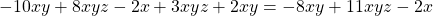Example Four (Eye Tester)

This example may hurt your eyes as the exponents are different for each of the three letter variables. If you are using paper, it would be a good idea to circle, underline or put some sort of tick marks for like terms.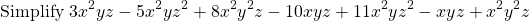This next line is putting the like terms together in their own groups.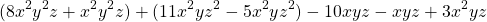It is now easier to simplify.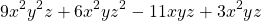Pixabay Image Source

### Examples Of Solving Equations With Like Terms

An extension of combining like terms is solving equations with like terms. As there are equations now, the equal sign is there.

Example One

Solve for x in the equation 3x - 4x + 7 = 10.

For this example, the goal is to isolate for +x. The first step is to combine like terms with the x variable terms and then use simple algebra to isolate for x.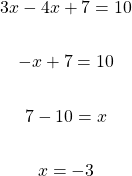Example Two

Solve for x in the equation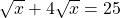.

The main idea here is to combine like terms, solve for the square root of x then raise each side to the power of 2 to obtain the value of x.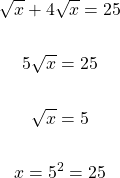Example Three

Solve for y in the equation y - 2x + 15 = -9x + 1.

The strategy here is to move everything that does not contain the variable y to the other side of y. Once that is done, combine like terms and simplify to obtain a result for y. Note that the quantity for y is not a number.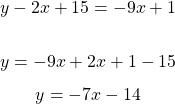Pixabay Image Source

### Practice Problems

Part One - Simplify These Algebraic Expressions

1. 2x -2 + 5x + 10

2. 3xy - x + 7xy - 8y + 2x

3.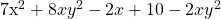4.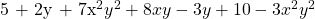Part Two - Solve For x

1. 3x - x + 3 = 7

2. 5x - 2 = 8x + 7

3.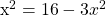4.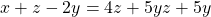### Solutions To Practice Problems

Part One

1. 7x + 8

2. 10xy + x - 8y

3.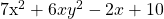4.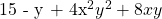Part Two

1. x = 2

2. x = -3

3. x = 2 or x = -2

4.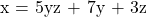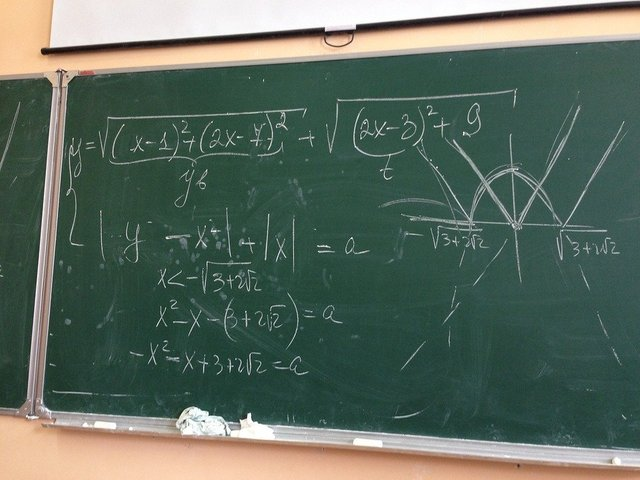Pixabay Image Source